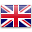# Interval

Sort by:
##### 1.5 時間到了! Time's Up!

STEM lessons + blocks

Students will solve word problems involving addition and...##### 1.6 房子牢固性測試 Build it up, break it down

STEM lessons + blocks

Students will investigate how a house can be...STEM lessons + blocks

Students will gain understanding of how technology can...##### 2.1 小夜燈 Night Light

STEM lessons + blocks

Students will gain understanding of how a product...##### 2.3 播種生長 Sow and Grow

STEM lessons + blocks

Students will gain understanding of how change occurs...##### 2.7 打鼓模擬器 Drum Simulator

STEM lessons + blocks

Students will gain understanding of how the speed...##### 3.1 物質的性質 Properties of Matter

STEM lessons + blocks

Students will gain understanding of how an object...##### 3.4 地震模擬器 Earthquake Simulator

STEM lessons + blocks

Students will gain understanding of what an earthquake...##### 3.6 燈塔 Lighthouse

STEM lessons + blocks

Students will investigate how light reflecting from objects...##### 3.8 熔岩燈 SAM Lava Lamp

STEM lessons + blocks

Students will gain understanding of water erosion and...##### 4.1 探索電路 Exploring Circuit

STEM lessons + blocks

Students will gain understanding of how electricity is...##### 4.10 比率 Ratio

STEM lessons + blocks

Students will work with collections of physical objects,...##### 4.3 黑夜與白晝 Night and Day

STEM lessons + blocks

Students will gain understanding of how the Earth...##### 4.4 摩斯密碼警報 Morse Code Alert

STEM lessons + blocks

Students will extend their knowledge of Morse Code,...##### 4.9 飲水機監控系統 Water Dispenser

STEM lessons + blocks

Students will gain understanding of the positive and...